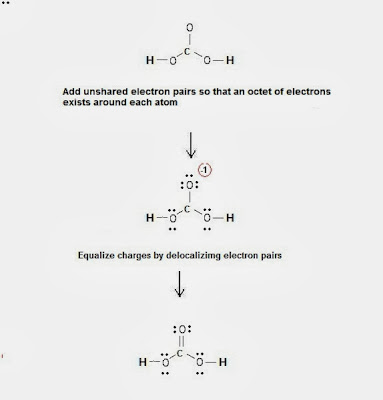Lewis dot structure of carbonic acid H2CO3 | Chemistry Net

# Lewis dot structure of carbonic acid H2CO3

A simple method for drawing Lewis structures: Carbonic Acid

# A simple method for drawing Lewis structures: Carbonic Acid

A simple method for writing Lewis structures (electron Lewis dot structures) was given in a previous post entitled Lewis Structures and the Octet Rule”.

Several worked examples relevant to this procedure were given in previous posts please see the post entitled "Lewis Structures & the Octet Rule - Theory & Examples"

Let us examine the case of carbonic acid H2CO3. How can we draw the carbonic acid Lewis dot structure?

Step 1: Connect the atoms with single bonds.

##### Fig. 1 : Connect the atoms of carbonic acid with single bonds

Step 2: Calculate the # of electrons in π bonds (multiple bonds) using  formula (1):

Where n in this case is 4 since H2CO3 consists of six atoms but two of them are H atoms.

Where V = (1 + 6 + 4 + 6 + 6 + 1) = 24

Therefore, P = 6n + 2 – V = 6 * 4 + 2 – 24 = 2      So  there are 2 π electrons in H2CO3and therefore 1 double bond must be added to the structure of Step 1.

Step 3 & 4: The Lewis structure of H2COis derived below:##### Fig. 2: Lewis structure of carbonic acid H2CO3

For a detailed description of the above method please see the following video:

Relevant Posts

Lewis Structures and the Octet Rule - A simple method to write Lewis Structures

Simple method for writing Lewis Structures – Ozone O3 and carbonate CO3-2 ion

Lewis dot structures - Electron dot structure of CO

Simple Method for drawing Lewis structures (Video): H2CO3

References

1. G.N. Lewis, J.A.C.S, 38, 762-785, (1916)
2.  E. C. McGoran, J. Chem. Educ., 68, 19-23 (1991)
3. A.B.P. Lever, J. Chem. Educ., 49, 819-821, (1972)

Key Terms

electron dot Lewis structures, Lewis Structures and the Octet Rule, how can we draw the carbonic acid Lewis dot structure